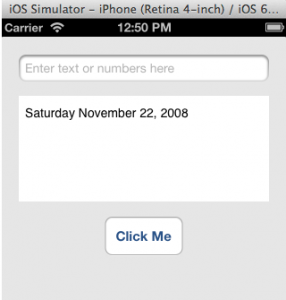The NSDate class is used for constructing and manipulating date and time objects within your iOS application. You can use it to do the following:

1. get today’s date and time
2. get the date and time in the past or future
3. calculate difference between dates
 To practice source code presented in this lesson, you should download and unzip this file.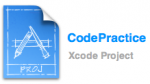Launch the project (CodePractice.xcodeproj) and enter code in the viewController.m file’s buttonTapped method, then click the “Click Me” button to execute the method’s code.

Now, the NSDateFormatter class is used for formatting NSDate object before displaying it to the user. In this tutorial you will learn how to use both classes. Let’s start by creating an NSDate object to store today’s date and time.

```// Create and initialize an NSDate object
NSDate *todaysDate = [NSDate date];
```

### The NSDateFormatter Class

Before you can display the date and time stored in the todaysDate object, you will have to format it with the NSDateFormatter class first. Say you wanted to display todaysDate like so:

Month Date, Year Hour:Minutes:Seconds (AM or PM)

you’ll need to used this code:

Code Output
```// Create and initialize an NSDate object
NSDate *todaysDate = [NSDate date];

// Create an NSDateFormatter object
NSDateFormatter *dateFormat = [[NSDateFormatter alloc] init];

// Set the date format
[dateFormat setDateFormat:@"MMMM d, yyyy hh:mm:ss a"];

// Create a string for storing the formatted date
NSString *todaysDateString;

// Format todaysDate as a string, then assign it to the todaysDateString object
todaysDateString = [dateFormat stringFromDate:todaysDate];

// Finally, display the formatted date and time in the UITextView control
self.outputBox.text = [@"Today's date is\n" stringByAppendingString:todaysDateString];
```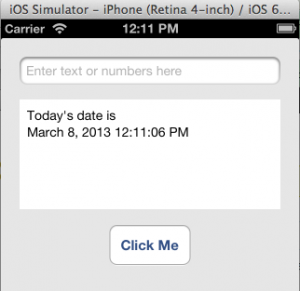#### Code Notes

You passed this format specifier to the setDateFormat: method to set the date format before displaying it in the UITextView control.

Above format specifier is defined by the Unicode standard that you can find here and they are interpreted like this:

 MMMM the full name of the month d the day of the month, with no leading zero yyyy the full four-digit year hh a two-digit hour (with leading zero if needed) mm a two-digits representing minutes ss two digits representing seconds a AM or PM

I’ve shown you how to get, format, and display today’s date. If you wanted to display the date only, you’d modify the setDateFormat: methods’s argument by removing these parts of the format specifier: hh:mm:ss a.

To display only the time, you’d modify the setDateFormat: method’s argument as well, by removing these parts: MMMM, d, yyyy, :ss.

Changing the number of specifiers of the setDateFormat: method’s argument, according to those shown below, changes the output.

### Display Past and Future Date

Say today’s date is Monday Sep 3, 2012 and you want to display yesterday’s date (Sun Sept 2, 2012) in the UITextView control. You would do it like this:

Code Output
```// Get the date for exactly 24 hours ago
NSDate *dateInThePass =[NSDate dateWithTimeIntervalSinceNow: -(24 * 60 * 60)];

// Create an NSDateFormatter object
NSDateFormatter *dateFormat = [[NSDateFormatter alloc] init];

// Set the pattern of how you want the date to display in the UITextView control
[dateFormat setDateFormat:@"EEE MMM d, yyyy"];

// Create a string for storing the formatted date
NSString *dateInThePassString;

// Format the date as a string, then assign it to the dateInThePassString object
dateInThePassString = [dateFormat stringFromDate:dateInThePass];

// Finally, display the formatted date in the UITextView control
self.outputBox.text = [@"Yesterday was\n" stringByAppendingString:dateInThePassString];
```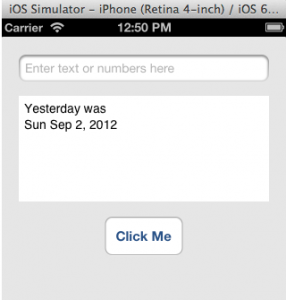##### How The Date is Calculated

You are probably wondering how the heck the app calculated the date shown in above output? Take a look at code line 02 above, specifically this part:
-(24 * 60 * 60)

It is responsible for calculating the date, one day in the past. Now let’s assume today’s date is Mon Sept 3, 2012. Base on this date, here is a break down of how the date calculation is done.

24 = hours in a day
60 = minutes
60 = seconds
* = multiplication

So (24 * 60 * 60) = 86400 seconds in a day. Now, adding the minus sign in front of (24 * 60 * 60) means we are subtracting a day in the past; thus giving us yesterday’s date:
Sun Sept 2, 2012

That’s how you calculate a date in the pass. Now to calculate a date in the future, you replace the minus sign with a plus sign like this:
+(24 * 60 * 60)

thus giving you tomorrow’s date, Tue Sept 4, 2012.

#### Calculating Specific Dates in The Past and Future

Now you know the basics of calculating a past and future date, how about calculating a date even further in the past or future. Well, you will have to expand the basic date calculation formula to what’s shown in this table. You should change the number for days in both formulas to what ever you want.

Past Date Calculation Formula Future Date Calculation Formula
// Subtract days, hours, minutes, and seconds in the future
double seconds = -(88*24*60*60);
// Add days, hours, minutes, and seconds in the future
double seconds = +(88*24*60*60);

Say today’s date is what’s shown in the September calendar below. You want the app to calculate and display the date for 45 days from that date, which is the date highlighted in the October calendar.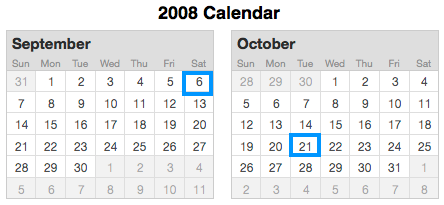Well, you’d have to change the button method’s code code shown below:

Code Output
```// Seconds to add
double totalSeconds = +(45*24*60*60);

// Get the date for exactly 45 days from now
NSDate *dateInTheFuture =[NSDate dateWithTimeIntervalSinceNow: totalSeconds];

// Create an NSDateFormatter object
NSDateFormatter *dateFormat = [[NSDateFormatter alloc] init];

// Set the pattern of how you want the date to display in the UITextView control
[dateFormat setDateFormat:@"EEE MMM d, yyyy"];

// Create a string for storing the formatted date
NSString *dateInTheFutureString;

// Format the date as a string, then assign it in the dateInTheFutureString object
dateInTheFutureString = [dateFormat stringFromDate:dateInTheFuture];

// Finally, display the formatted date in the UITextView control
self.outputBox.text = [@"45 days from now the date will be\n" stringByAppendingString:dateInTheFutureString];
```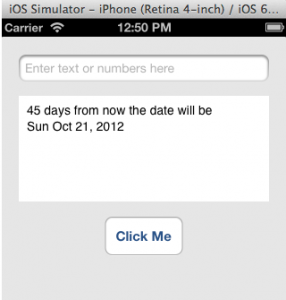### Calculate Difference Between Dates

Say you wanted to calculate the number of days there are between these two dates.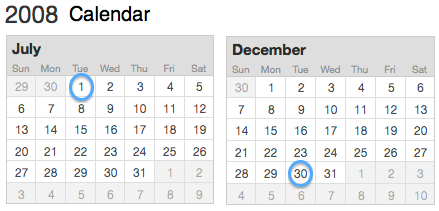Further more, you want to display the calculation result in the UITextView control. You’d have to do the following:

1. Declare an NSDateFormatter object
2. Convert both dates to NSDate objects
3. Calculate difference between NSDate objects
4. Divide calculation result by the number of seconds in a day (86400)
5. Format step 4 calculation result, then display it in the UITextView control

Here is the code to do above steps:

Code Output
```// Step 1: Declare and set an NSDateFormatter object
NSDateFormatter *dateFormat = [[NSDateFormatter alloc] init];
[dateFormat setDateFormat:@"yyyyMMdd"];

// Step 2: Convert both dates to NSDate objects
NSDate *dateObject1 = [dateFormat dateFromString:@"20080701"];
NSDate *dateObject2 = [dateFormat dateFromString:@"20081230"];

// Step 3: Calculate difference between NSDate objects
NSTimeInterval secondsBetweenDates = [dateObject2 timeIntervalSinceDate:dateObject1];

// Step 4: Divide calculation result by number of seconds in a day (86400)
int numberOfDays = secondsBetweenDates / 86400;

// Step 5: Format step 4 calculation result, then display it in the UITextView control
self.outputBox.text = [NSString stringWithFormat:
@"There are %d days between the two dates.",
numberOfDays];
```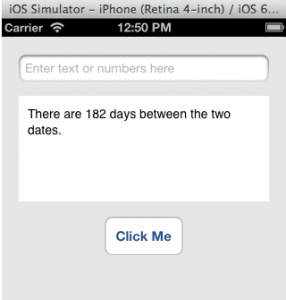#### Code Notes

Take a look at code line 03: [dateFormat setDateFormat:@”yyyyMMdd”];
The setDateFormat: method’s argument sets up the date pattern matching the pattern of string date shown in code lines 06 and 07. Now, if you wanted to see the value stored in the secondsBetweenDates variable, just enter this code right after code line 18.

`self.outputBox.text = [NSString stringWithFormat:@"%f\n",secondsBetweenDates];`
##### How the date calculation was done?

You are probably wondering how the date calculation was done? Well, wonder no more because I’ll explain exactly how. This calendar highlights the date range stored in these variables: dateObject1 and dateObject2-see code lines 06 and 07 above.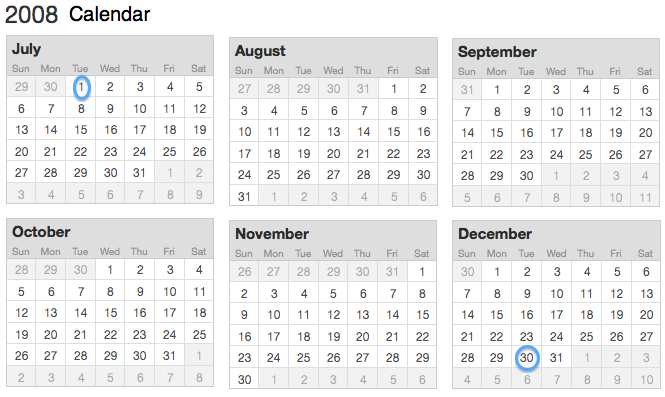Now, here is how you go about calculating the number of days between dates highlighted in above calendar. Start by jotting down the months and number of days from above calendar like this: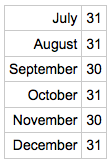Now, minus 1 day from July and December. Your note should now look like this: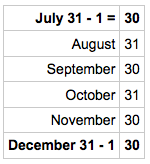The last thing you need to do is line up the numbers and sum them, to get the numbers of days between 20080701 – 20081230, which should equal 182.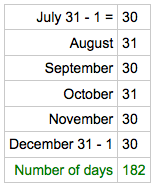That’s pretty much how you calculate the number of days between dates shown in above calendar. Now these code lines:

```NSTimeInterval secondsBetweenDates = [dateObject2 timeIntervalSinceDate:dateObject1];
int numberOfDays = secondsBetweenDates / 86400;
```

are responsible for doing exactly what you did by hand. The message to the right of the equal sign in the first statement counts the number of seconds between 20080701 and 20081230 and place it in the secondsBetweenDates variable. The second statement divides the secondsBetweenDates by the number of seconds a day have, then assign it in the numberOfDays variable. Here is the translation of the code:

182 = 15728400.000000 / 84600

By the way, NSTimeInterval’s data type is of double; therefor, the value stored in the secondsBetweenDates variable is 15728400.000000.

### Convert a String Date to an NSDate Object

Say you wanted to convert the date shown in this calendar to an NSDate object.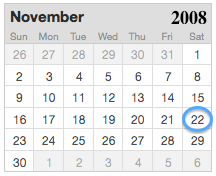You do it like this:

```// Place the string date in an NSString object
NSString *stringDate = @"2008-11-22";

// Declare an NSDateFormatter object
NSDateFormatter *dateFormat = [[NSDateFormatter alloc] init];

// Set the pattern of the stringDate object
[dateFormat setDateFormat:@"yyyy-MM-dd"];

// Convert the stringDate object to an NSDate object
NSDate *dateObject = [dateFormat dateFromString:stringDate];
```

#### Display NSDate Object in The UITextView Control

Now that you’ve converted a string date to an NSDate object, you probably want to display it in the UITextViewControl like this:

Saturday November 22,2008

Before you display the NSDate object in the UITextView control, you’d have to set up the display pattern and convert the date object to a string first. Here is the code to do it:

Code Output
```// Set the date pattern
[dateFormat setDateFormat:@"EEEE MMMM d, YYYY"];

// Convert the dateObject to a string, then assign it to the UITextView control
self.outputBox.text = [dateFormat stringFromDate:dateObject];
```